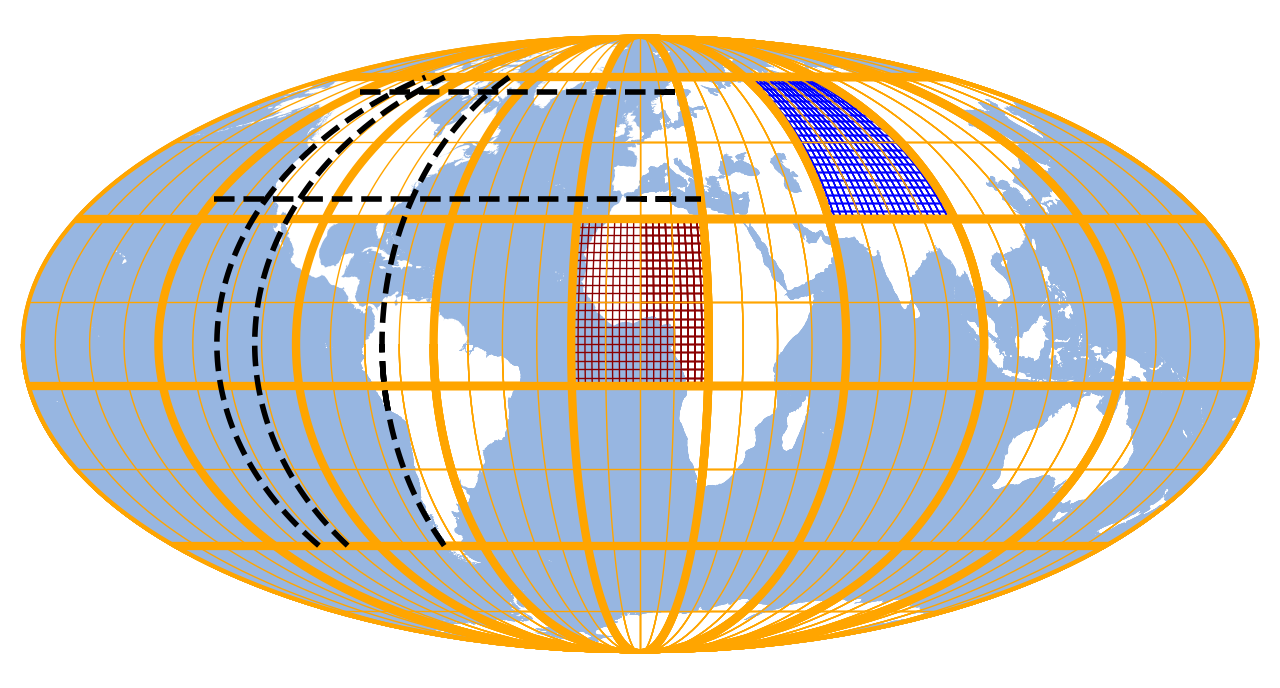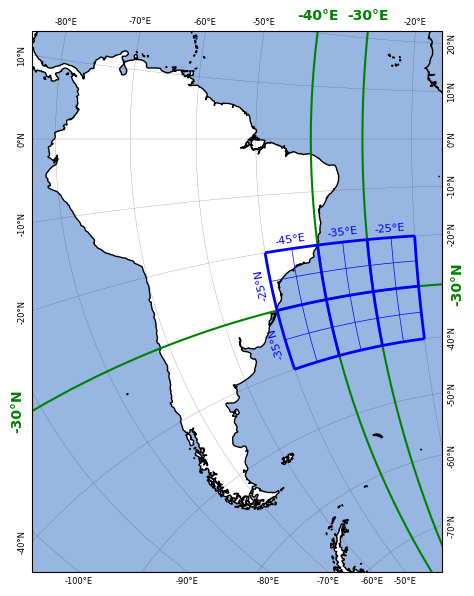# ▦ Gridlines

## How to add grid lines to a map

Gridlines can be added to the map via `Maps.add_gridlines()`.

If `d` is provided, the gridlines will be fixed

• If you provide a number, it is used as grid-spcing (in degrees)

• If you provide a `list` or `numpy.array`, it is used to draw lines only at the specific coordinates

• To use different settings for latitude and longitude lines, provide a `2-tuple` of the aforementioned types.

If no explicit grid-spacing is provided (e.g. `d=None`), the grid is dynamically updated based on the visible extent.

• Use `auto_n=...` to adjust the density of the auto-gridlines.

 `Maps.add_gridlines` Add gridlines to the map.
 `Maps.add_gridlines` Add gridlines to the map.
 ```from eomaps import Maps m = Maps(Maps.CRS.Mollweide(), frameon=False) m.add_feature.preset.ocean() # add gridlines with a fixed grid-spacing mg = m.new_layer("grid") g0 = mg.add_gridlines(d=40, ec="orange", lw=3, zorder=2) g1 = mg.add_gridlines(d=(10, 20), ec="orange", lw=.5, zorder=1) # add fine-grained gridlines in a specific area g2 = mg.add_gridlines(d=2, ec="darkred", lw=0.5, zorder=0, bounds=(-20, 20, -10, 30)) g3 = mg.add_gridlines(d=2, ec="b", lw=0.5, zorder=0, bounds=(60, 100, 30, 70)) # add dedicated gridlines at specific coordinates g4 = mg.add_gridlines(([-123, -112, -75], [35, 65]), ec="k", lw=2, ls="--", zorder=20, bounds=(-140, 20, -50, 70) ) m.show_layer(m.layer, "grid") ```In addition, the returned `GridLines` instance supports the following useful methods:

 `GridLines` Class to draw grid-lines. `GridLines.set_d` Set a fixed gridline distance (in degrees). `GridLines.set_auto_n` Set the number of (auto) gridlines to draw in the currently visible extent. `GridLines.set_n` Set the number of intermediate points to calculate for each gridline. `GridLines.set_bounds` Set the extent of the area in which gridlines are drawn. `GridLines.update_line_props` Set/update the properties of the drawn lines (e.g. color, linewidth etc.). `GridLines.remove` Remove the grid from the map. `GridLines.add_labels` Add labels to the gridlines.

## How to add labels to a grid

Labels can be added to a grid via the `GridLines.add_labels()` directive.

In general, labels are added at points where the lines of the grid intersects with the axis-boundary. (Note that this provides a lot of flexibility since a map can have as many grids as you like and each grid can have its own labels!)

The `where` parameter can be used to control where grid labels are added:

• Use an arbitrary combination of the letters `"tblr"` to draw labels at the top, bottom, left or right boundaries.

• If this option is used, longitude-lines are only labeled top/bottom and latitude-lines are only labeled left/right.

• Use `"all"` to label all intersection points.

• Use an integer to draw labels only at the nth found intersection-points.

In addition, the `exclude` parameter can be used to exclude specific labels based on their lon/lat values and the `every` parameter can be used to add a label only to every nth grid line.

To change the appearance of the labels, any kwarg supported by matplotlib.pyplot.text can be used (e.g. color, fontsize, fontweight, …).

 ```from eomaps import Maps m = Maps(Maps.CRS.Stereographic(), figsize=(5, 6)) m.set_extent((-83, -20, -59, 13)) m.add_feature.preset.coastline() m.add_feature.preset.ocean() # draw a regular grid with 10 degree grid-spacing # and add labels to all lines except some selected lines g = m.add_gridlines(10, lw=0.25, alpha=0.5) g.add_labels(fontsize=6, exclude=([-40, -30], [-30])) # draw some specific gridlines and add bold green labels g = m.add_gridlines(([-40, -30], [-30]), c="g", lw=1.5) gl0 = g.add_labels(where="tlr", c="g", offset=15, fontweight="bold") # draw a bounded grid and add labels g = m.add_gridlines(10, bounds=[-50, -20, -40, -20], c="b", lw=2) g = m.add_gridlines(5, bounds=[-50, -20, -40, -20], c="b") gl = g.add_labels(where=0, fontsize=8, every=(1, -1, 2), c="b") ```